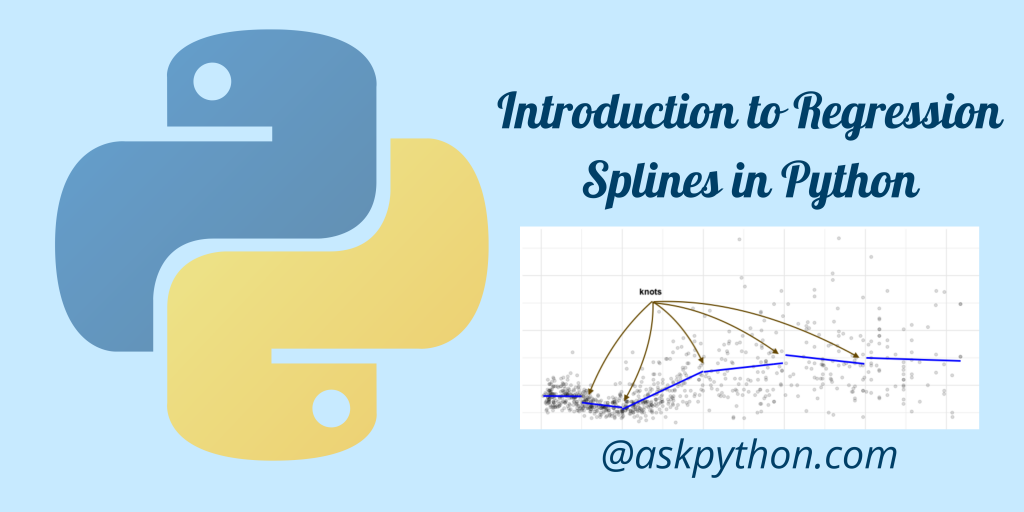# Regression Splines in Python – A Beginners IntroductionHey coder! I am sure you have heard about Linear regression which is one of the simplest algorithms that teaches a lot about the relationship between dependent and non-dependent variables.

The result generally comes as a straight line. The problem is that in practical scenarios, having a straight line is not always possible.

To overcome that we have the polynomial curves (smooth curves). But the polynomial curves can get super complex and hence are avoided.

To even overcome this drawback, in this tutorial, I will introduce you to regression splines available in Python.

Also Read: Logistic Regression – Simple Practical Implementation

In order to create a spline regression, the whole dataset is divided into smaller bins. And the regression line is predicted for each bin and the separate lines are joined together by knots.

Now that we are clear with how regression spline works, let us move to the code implementation of the same in the Python programming language.

## Implementing Regression Splines in Python

Let us first download the dataset for the tutorial. The dataset can be downloaded here. The dataset is about the wages of people along with a lot of information about the people getting paid.

We will be loading the dataset using the `read_csv` function of the pandas module in Python.

```import pandas as pd
df
```

Let’s have a look at what the dataset looks like in the image below.

### 2. Creating X and Y values

To understand the spline plots better, we will have a look at two columns that don’t have any direct relation between them. Let’s have a look at the relation between the age and wage of a person.

The age won’t directly influence the wage of a person and hence will help us understand its implementation better.

```X = df[['age']]
y = df[['wage']]
```

### 3. Splitting the data into train and test data

The next step is to split the data into training and testing datasets using the 80:20 rule where 80% of the data is used for training and the rest 20% is set for testing the model.

```from sklearn.model_selection import train_test_split
X_train, X_test, y_train, y_test = train_test_split(X, y, test_size=0.2, random_state = 1)
```

### 4. Data Visualization

In this step, let’s visualize the initial dataset that we just created using the code below. We will visualize both the testing and training dataset.

```import matplotlib.pyplot as plt
import seaborn as sns
sns.set_theme(style="ticks", rc={"axes.spines.right": False, "axes.spines.top": False})

plt.figure(figsize=(10,8))
sns.scatterplot(x=X_train['age'], y=y_train['wage'], color="red",alpha=0.2)
plt.title("Age vs Wage Training Dataset")

plt.figure(figsize=(10,8))
sns.scatterplot(x=X_test['age'], y=y_test['wage'], color="green",alpha=0.4)
plt.title("Age vs Wage Testing Dataset")

plt.show()
```

The resulting plots are shown below.

### 5. Applying Linear Regression on the Dataset

Applying linear regression to the dataset is simple if you have implemented it before. We will also be computing the mean squared error of the model using the training dataset.

```from sklearn.linear_model import LinearRegression
lm = LinearRegression()
lm.fit(X_train,y_train)

print("Slope of the Regression Line is : ", lm.coef_)
print("Intercept of Regression Line is : ",lm.intercept_)

from sklearn.metrics import mean_squared_error
pred_test = lm.predict(X_test)
rmse_test =mean_squared_error(y_test, pred_test, squared=False)

print("Accuracy of Linear Regression on testing data is : ",rmse_test)
```

The results for the model came as below.

```Slope of the Regression Line is :  [[0.68904221]]
Intercept of Regression Line is :  [82.09009765]
Accuracy of Linear Regression on testing data is :  40.68927607250081
```

Now, let’s plot the regression line for the dataset using the code below.

```plt.figure(figsize=(10,8))
sns.regplot(x=X_test['age'], y=y_test['wage'], ci=None, line_kws={"color": "red"})
plt.title("Regression Line for Testing Dataset")
plt.show()
```

### 6. Applying Polynomial Regression

Let’s try to fit polynomial regression into the dataset using the code below and see if we can increase the accuracy to some extent.

```from sklearn.preprocessing import PolynomialFeatures
poly = PolynomialFeatures(2)

X_train_poly = poly.fit_transform(X_train)
X_test_poly = poly.fit_transform(X_test)
pm = LinearRegression()
pm.fit(X_train_poly,y_train)

pred_test = pm.predict(X_test_poly)
rmse_test =mean_squared_error(y_test,pred_test,squared=False)

print("Accuracy of Polynomial Regression on testing data is : ",rmse_test)
```

We can also plot the polynomial regression line using the code below.

```plt.figure(figsize=(10,8))
sns.regplot(x=X_test['age'], y=y_test['wage'], ci=None, line_kws={"color": "red"},order=2)
plt.title("Polynomial Regression Line for Testing Dataset")
plt.show()
```

### 7. Implementation of Cubic Spline

Implementation and plotting of cubic spline are very similar to the previous implementations. It won’t be difficult to understand the code below.

```from patsy import dmatrix
transformed_x = dmatrix("bs(train, knots=(25,40,60), degree=3, include_intercept=False)",
{"train": X_train},return_type='dataframe')
import statsmodels.api as sm
cs = sm.GLM(y_train, transformed_x).fit()
pred_test = cs.predict(dmatrix("bs(test, knots=(25,40,60), include_intercept=False)",
{"test": X_test}, return_type='dataframe'))
rmse_test =mean_squared_error(y_test, pred_test, squared=False)
print("Accuracy for Cubic Spline on testing data is : ",rmse_test)

import numpy as np
plt.figure(figsize=(10,8))
xp = np.linspace(X_test.min(),X_test.max(), 100)
pred = cs.predict(dmatrix("bs(xp, knots=(25,40,60), include_intercept=False)",
{"xp": xp}, return_type='dataframe'))
sns.scatterplot(x=X_train['age'], y=y_train['wage'])
plt.plot(xp, pred, label='Cubic spline with degree=3 (3 knots)', color='red')
plt.legend()
plt.title("Cubic Spline Regression Line for Testing Dataset")
plt.show()
```

The results are shown below.